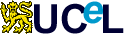Reusable Learning Object

# Power and sample size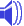Testing hypotheses

A hypothesis test is when a researcher compares two means or two proportions and conducts a statistical analysis based on two opposing hypotheses.

The null hypothesis,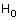, states there is no difference between the two groups. The alternative hypothesis,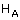, is the view that there is a difference.

This table shows the possible outcomes of a hypothesis test in relation to the true situation. The true situation, which is unknown to the researcher, is that the null hypothesis is either true or false. If the researcher decides to reject the null hypothesis when it is false or not to reject the null hypothesis when it is true then they will make the correct decision. On the other hand, if the researcher rejects the null hypothesis when it is true they will make a type I error. The probability of making a type I error is determined by the significance level and if the null hypothesis is true the probability of making a type I error is equal to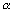. The researcher will also make an error if they fail to reject a false null hypothesis; this is a type II error and has a probability of occurring equal to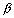or 1-power of the study.

section --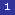-- 2 -- 3 -- 4 -- 5 -- 6 -- resources
------------------------------------
Page created: 1 September, 2002
By: Dawn Leeder
Last updated: 25 June, 2003 4:06 PM
By: Alan Leeder